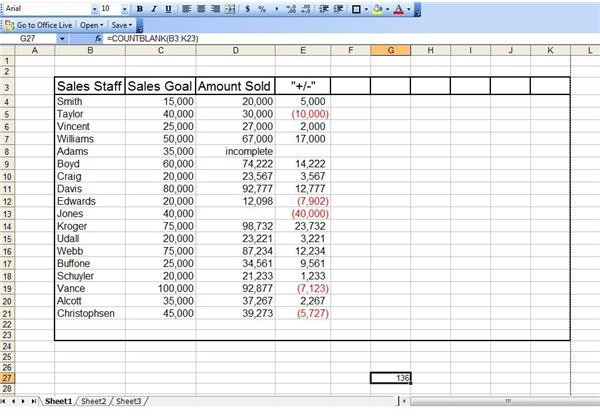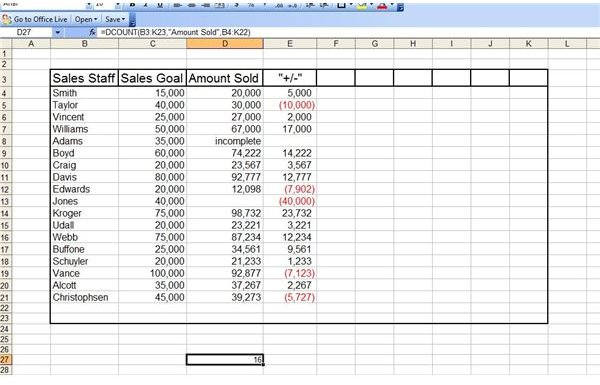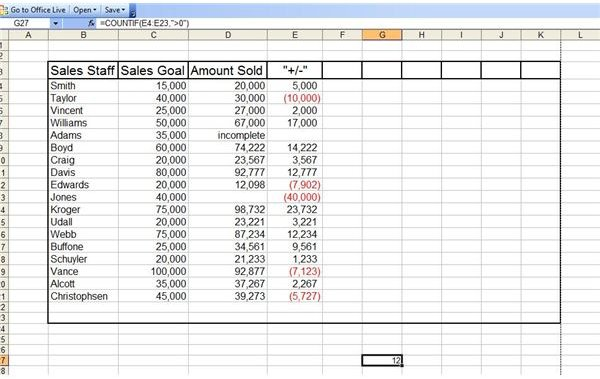# How to Use the Excel COUNT Function

Page content

As with many Excel functions, there are several variations of the COUNT function. Read on to learn which one best suits your need.

## COUNT

Excel’s basic COUNT function counts the number of cells in a range that contain numerical data. This can be helpful if you have an extensive spreadsheet and you want to make sure no cells that should contain numbers were missed. The syntax for Excel’s COUNT function is COUNT(value1,value2,…). To apply the COUNT function, select the cell in which you want the total number of cells to appear. Go to the Function Bar and enter =count( and then select the range of cells you want counted. Press Enter.

## COUNTBLANK

Excel’s COUNTBLANK function acts in almost an opposite manner of its COUNT equivalent. COUNTBLANK will count the number of cells in a range that are empty. This function can be extremely helpful if you have a large Excel spreadsheet that should not contain any blanks. The syntax for COUNTBLANK is also extremely uncomplicated. It is COUNTBLANK(range).

To use Excel’s COUNTBLANK function, start by selecting the cell in which you want the result to appear and go to the Formula Bar. Enter =countblank( and then select the range you want counted. Press Enter and the total number of blank cells will appear in the cell.## DCOUNT

DCOUNT is a variation of Excel’s COUNT function. The DCOUNT function will count cells containing numbers in an Excel database table, but only if those cells meet criteria that you determine. The syntax for the DCOUNT function is DCOUNT(database,field,criteria). To use the DCOUNT function, start by selecting the cell in which you want the count result to appear.

For our example, we want to know the number of cells in the database “Amount Sold” fields contain numbers. Therefore, the formula we enter will be =DCOUNT(B3:K23,“Amount Sold”,B4:K22)## COUNTIF

The COUNTIF function in Excel works much like DCOUNT, only you can apply it to a single column or smaller range of cells than an entire database table. The syntax for this function is COUNTIF(range,criteria).

For our example, let’s say we want to know how many members of the sales staff team reached or exceeded their goals for the quarter. To determine this, we will want to know how many cells in the “+/-” column contain positive numbers. The formula we will use is =COUNTIF(E4:E23,">0")

It is important to remember to use the quotation marks if your criteria are anything besides basic numeric amounts.For help on other Excel functions, read Using the IF Function in Excel 2007 and Microsoft Excel’s MATCH Function.